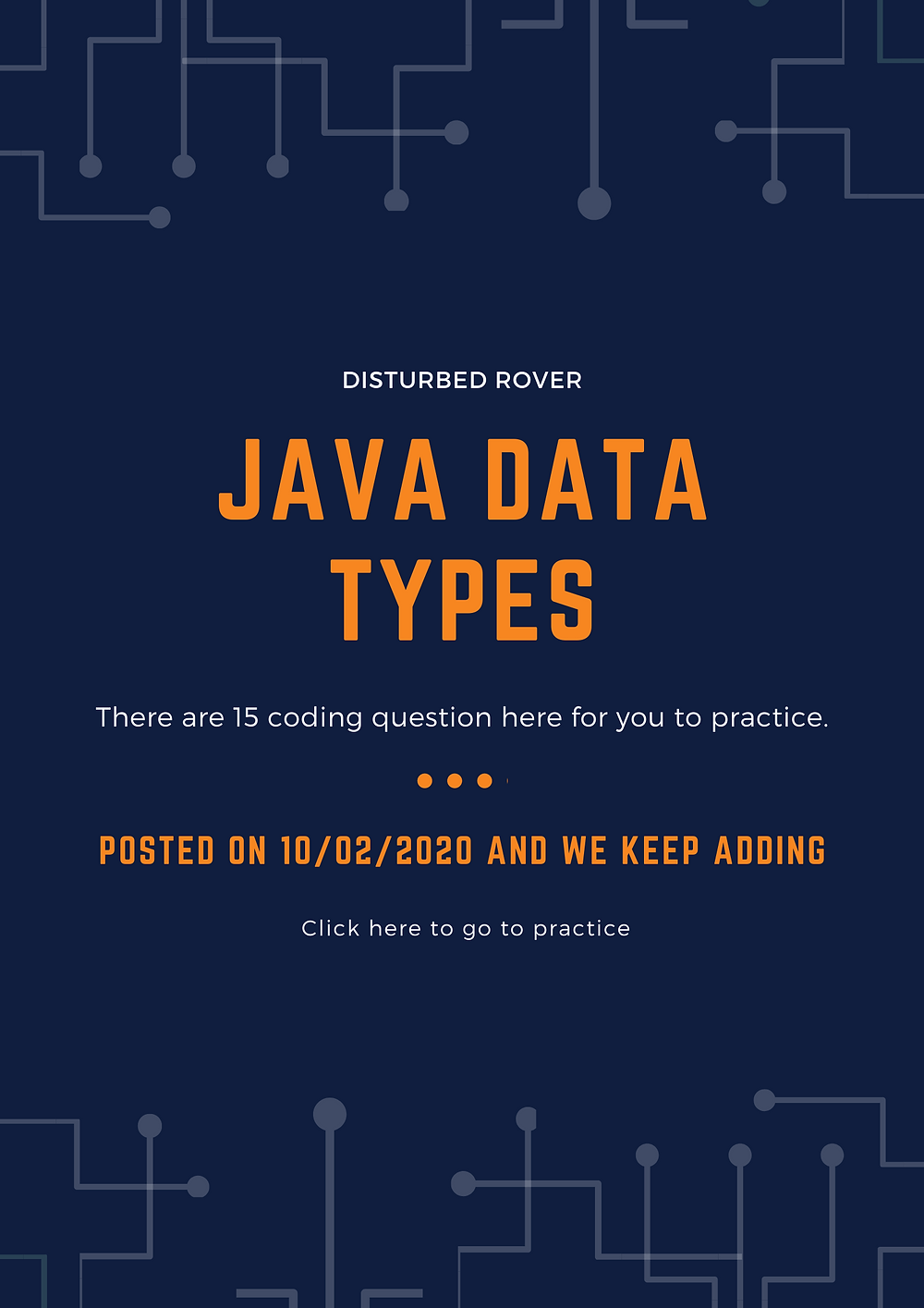Search

# Data Types Problems(JAVA)

Here I have collected 15 to 20 questions which are based on solely on java data types, although I am writing this blog you can find this question on the net, I have collected for you

to practice. Also, there is a code editor is embedded for you to test the code.Let's begin with the first question:-

### 1. Write a Java program to convert temperature from Fahrenheit to Celsius degree.

Test Data

Input a degree in Fahrenheit: 212

Expected Output:

212.0 degree Fahrenheit is equal to 100.0 in Celsius

### 2. Write a Java program that reads a number in inches, converts it to meters.

Note: One inch is 0.0254 meters.

Test Data

Input a value for inch: 1000

Expected Output :

1000.0 inch is 25.4 meters

### 3. Write a Java program that reads an integer between 0 and 1000 and adds all the digits in the integer.

Test Data Input an integer between 0 and 1000: 56 Expected Output : The sum of all digits in 565 is 16

### 4. Write a Java program to convert minutes into a number of years and days.

Test Data Input the number of minutes: 3456789 Expected Output : 3456789 minutes is approximately 6 years and 210 days

### 5. Write a Java program that prints the current time in GMT.

Test Data Input the time zone offset to GMT: 256 Expected Output: Current time is 23:40:24

### 6. Write a Java program to compute body mass index (BMI).

Test Data Input weight in pounds: 452 Input height in inches: 72 Expected Output: Body Mass Index is 61.30159143458721

### 7. Write a Java program to takes the user for a distance (in meters) and the time was taken (as three numbers: hours, minutes, seconds), and display the speed, in meters per second, kilometers per hour, and miles per hour (hint: 1 mile = 1609 meters).

Test Data Input distance in meters: 2500 Input hour: 5 Input minutes: 56 Input seconds: 23 Expected Output : Your speed in meters/second is 0.11691531 Your speed in km/h is 0.42089513 Your speed in miles/hr is 0.26158804

### 8. Write a Java program that reads a number and display the square, cube, and fourth power.

Expected Output:

Square: .2f

Cube: .2f

Fourth power: 50625.00

### 9. Write a Java program that accepts two integers from the user and then prints the sum, the difference, the product, the average, the distance (the difference between integer), the maximum (the larger of the two integers), the minimum (smaller of the two integers).

Test Data Input 1st integer: 25 Input 2nd integer: 5 Expected Output : Sum of two integers: 30 A difference of two integers: 20 Product of two integers: 125 Average of two integers: 15.00 A distance of two integers: 20 Max integer: 25 Min integer: 5

### 10. Write a Java program to break an integer into a sequence of individual digits.

Test Data Input six non-negative digits: 123456 Expected Output : 1 2 3 4 5 6

### 12. Write a Java program to compare two given signed and unsigned numbers.

13. Write a Java program to compute the floor division and the floor modulus of the given dividend and divisor.

### 16.What should be the output.

```class Main {
public static void main(String args[]) {
int t;
System.out.println(t);
}
}```

17. What should be the output.

```class Test {
public static void main(String[] args) {
for(int i = 0; 0; i++)
{
System.out.println("Hello");
break;
}
}
}  ```

### 18. What should be the output.

```class Test
{
public static void main(String[] args)
{
Double object = new Double("2.4");
int a = object.intValue();
byte b = object.byteValue();
float d = object.floatValue();
double c = object.doubleValue();

System.out.println(a + b + c + d );

}
} ```

Try all this code in this editor.

### Code Editor:-

Fun problem - How you can extract a set of URLs from the given email body?

123 views

See All